Example Questions

Example Question #6 : How To Find X Or Y Intercept

A line has the equation: 2x+4y=8.

What is the x-intercept?

4

-4

8

-8

0

4

Explanation:

To find the x-intercept, rearrange the equation 2x+4y=8 so that x is isolated:

2x=-4y+8

x=-2y+4

Using the point-slope formula, we see that the x-intercept is 4.

Example Question #7 : How To Find X Or Y Intercept

What is the y intercept of the following function of x?

y = 3x

3

–3

0

1

–1

0

Explanation:

The answer is 0 because in slope intercept form, y = mx + b; b is the y intercept. In this case b = 0.

Example Question #8 : How To Find X Or Y Intercept

What is the x-intercept of a line with a slope of 5 and y-intercept of 3.5?

(–0.7, 0)

(3.5, 0)

(0.7, 0)

(–3.5, 0)

(0, –0.7)

(–0.7, 0)

Explanation:

To solve this, first find the equation of our line.  The form of the question gives it to us very directly.  We can use the slope-intercept form (y = mx + b).

y = 5x + 3.5

The x-intercept is found by setting y = 0, because that will give us the x-value at which the line crosses the x-axis.

0 = 5x + 3.5; –3.5 = 5x; x = –3.5 / 5 or –0.7.  The point will be (–0.7, 0)

Example Question #9 : How To Find X Or Y Intercept

Determine the y-intercept of the following line: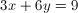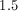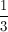Explanation:

The y-intercept occurs when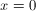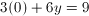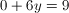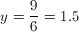Example Question #10 : How To Find X Or Y Intercept

At what point does the graph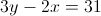cross the-axis?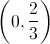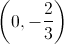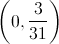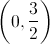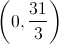Explanation:

The graph crosses the-axis where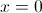. So plugging in and solving yields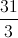Example Question #11 : How To Find X Or Y Intercept

Find the x-intercepts of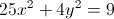.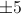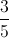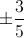Explanation:

To find the x-intercepts, plug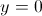into the equation and solve for.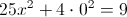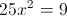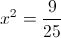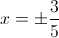Don't forget that there are two solutions, both negative and positive!

Example Question #12 : How To Find X Or Y Intercept

A line with the exquation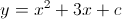passes through the point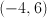.  What is the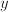-intercept?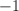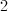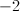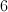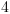Explanation:

By plugging in the coordinate, we can figure out that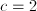.  The-Intercept is when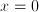, plugging in 0 forgives us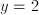.

Example Question #13 : How To Find X Or Y Intercept

What are the-intercept(s) of the following line: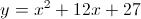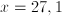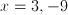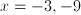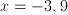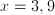Explanation:

We can factorand setequal to zero to determine the-intercepts.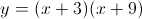satisfies this equation.

Therefore our-intercepts are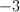and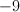.

Example Question #171 : Geometry

Which of the following lines does not intersect the line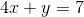?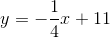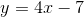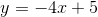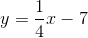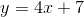Explanation:

Parallel lines never intersect, so you are looking for a line that has the same slope as the one given. The slope of the given line is –4, and the slope of the line in y = –4x + 5 is –4 as well. Since these two lines have equal slopes, they will run parallel and can never intersect.

Example Question #172 : Geometry

Find the y-intercept of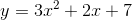.

3

14

12

5

7